Author: Oscar Cronquist Article last updated on March 19, 2019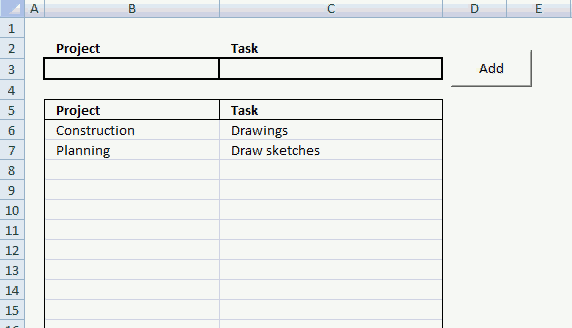In this small tutorial, I am going to show you how to create basic data entry with a small amount of VBA code.

Cell B3 and C3 are input cells. When you click button "Add", the data in cell B3 and C3 are copied to the first empty row in the list.

The last step in the code removes the values in cell B3 and C3, see the animated image above.

### Macro

```Sub AddText()
Dim Lrow As Single

With Worksheets("Sheet1")
Lrow = .Range("B" & Rows.Count).End(xlUp).Row + 1
.Range("B" & Lrow & ":C" & Lrow) = .Range("B3:C3").Value
.Range("B3:C3").Value = ""
End With

End Sub
```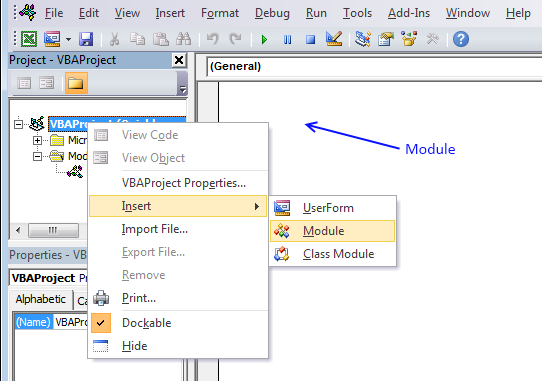1. Press Alt+F11 to open the VB Editor.
2. Right-click on your workbook name in the Project Explorer window.
3. Click "Insert".
4. Click "Module" to insert a code module to your workbook.
5. Copy macro and paste to code module, see image above.

### Explaining macro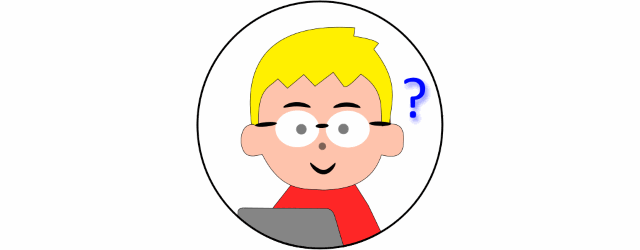#### Step 2 - Declare variables and data types

Dim Lrow As Single

#### Step 3 - Find last non-empty cell in column B

This line returns the row number of the last non-empty cell in column B:

Lrow = Worksheets("Sheet1").Range("B" & Rows.Count).End(xlUp).Row

We want the first empty cell so we add one to the code.

Lrow = Worksheets("Sheet1").Range("B" & Rows.Count).End(xlUp).Row + 1

This line of code saves the value to variable Lrow. In the example given above, the code saves the value 8 to Lrow.

#### Step 4 - Copy values

Let's use the value in Lrow and copy values from cell B3:C3 to the first empty row.

Worksheets("Sheet1").Range("B" & Lrow & ":C" & Lrow) = Worksheets("Sheet1").Range("B3:C3").Value

#### Step 5 - Remove values

The following line deletes the values in cell range B3:C3.

Worksheets("Sheet1").Range("B3:C3").Value = ""

End Sub

### Simplify code

Worksheets("Sheet1") is repeated many times. The WITH and WITH END statement allows you to simplify the code.

Dim Lrow As Single
With Worksheets("Sheet1")
Lrow = .Range("B" & Rows.Count).End(xlUp).Row + 1
.Range("B" & Lrow & ":C" & Lrow) = .Range("B3:C3").Value
.Range("B3:C3").Value = ""
End With
End Sub

### Create button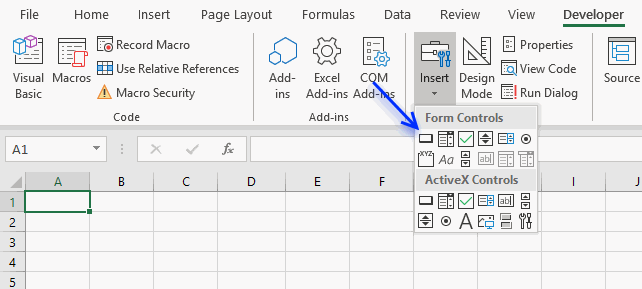1. Go to the Developer tab.
2. Click "Insert" button on the ribbon.
3. Click "Button" (Form Control).
4. Press and hold with left mouse button on the worksheet.
5. Drag with mouse to create the button then release the mouse button.

### Assign macro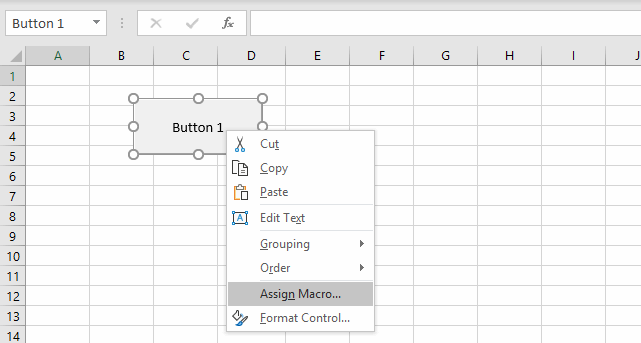1. Right click on your new button.
2. Assign a macro.